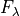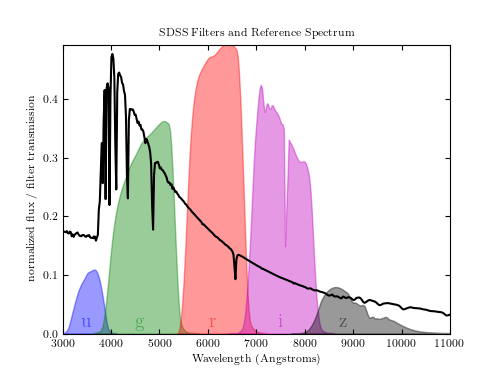# SDSS Filters¶

Figure C.1

The five SDSS filters, showing the total transmission taking into account atmospheric transmission and instrumental effects such as CCD efficiency. Shown for reference is the spectrum () of a star similar to Vega (alpha-Lyr), which for many years was used as a reference flux for magnitude calibration.# Author: Jake VanderPlas
#   The figure produced by this code is published in the textbook
#   "Statistics, Data Mining, and Machine Learning in Astronomy" (2013)
#   To report a bug or issue, use the following forum:
from matplotlib import pyplot as plt
from astroML.datasets import fetch_sdss_filter, fetch_vega_spectrum

#----------------------------------------------------------------------
# This function adjusts matplotlib settings for a uniform feel in the textbook.
# Note that with usetex=True, fonts are rendered with LaTeX.  This may
# result in an error if LaTeX is not installed on your system.  In that case,
# you can set usetex to False.
if "setup_text_plots" not in globals():
from astroML.plotting import setup_text_plots
setup_text_plots(fontsize=8, usetex=True)

#------------------------------------------------------------
# Set up figure and axes
fig = plt.figure(figsize=(5, 3.75))

#----------------------------------------------------------------------
# Fetch and plot the Vega spectrum
spec = fetch_vega_spectrum()
lam = spec
spectrum = spec / 2.1 / spec.max()
ax.plot(lam, spectrum, '-k')

#------------------------------------------------------------
# Fetch and plot the five filters
text_kwargs = dict(ha='center', va='center', alpha=0.5, fontsize=14)

for f, c, loc in zip('ugriz', 'bgrmk', [3500, 4600, 6100, 7500, 8800]):
data = fetch_sdss_filter(f)
ax.fill(data, data, ec=c, fc=c, alpha=0.4)
ax.text(loc, 0.02, f, color=c, **text_kwargs)

ax.set_xlim(3000, 11000)

ax.set_title('SDSS Filters and Reference Spectrum')
ax.set_xlabel('Wavelength (Angstroms)')
ax.set_ylabel('normalized flux / filter transmission')

plt.show()